# College GPA Calculator

GPA calculator helps you to calculate your school or college grade point average online.

##### More Useful Tools

College GPA Calculator is an online web application that helps you to calculate your college, high school, or cumulative grade point average. Also, it's a free and easy-to-use tool. Just select the grades and enter credit hours and get the results within a second.

Therefore, no need to do the manual calculation. You can easily figure out your GPA with just a few keystrokes.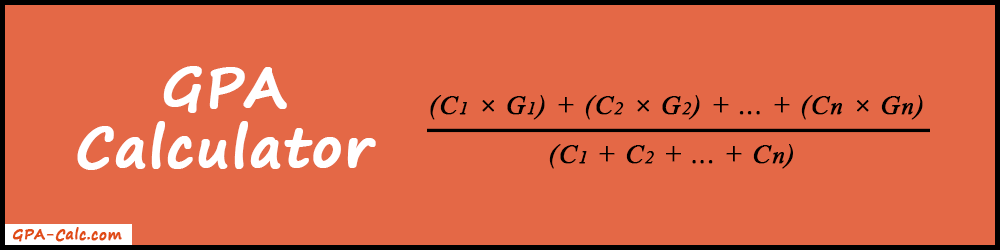In this article, you will learn about the GPA scale table, how to calculate GPA, and how to use the GPA Calculator. So, stay tuned and keep reading till the end.

#### What is GPA?

In real life, we heard this term many times when we are in school or college. So, let's understand it in-depth.

GPA stands for "Grade Point Average". It is a standard way of measuring achievements in school or college.

In other words, it is an average number that shows what you have scored in your classes throughout the term, semester, and year.

Mostly, the grade point average is calculated on a 4.0 scale. So, it may count in the range from 0.0 to 4.0.

0.0 defines the lowest score, and 4.0 defines the highest score. In addition, this type of GPA is known as an "Unweighted GPA".

But some academics consider Advanced Placement(AP) or Honors classes for extra points. So, they consider 5.0 as the highest score and it is known as a "Weighted GPA".

### Letter Grade to GPA Scale Table

In the following table, the letter grades are converted into numerical values. Also, we can call it "Grade Points". We need this table when we are calculating the GPA manually. It will help us to find the Grade Points from Letter Grades. Also, you will get more ideas about this table when we take some examples.

But you don't need to remember this table all the time. Our GPA calculator will automatically take the grade point values from the table. Therefore, you don't need to enter it manually.

A+ 97-100 4.33
A 93-96 4.00
A- 90–92 3.67
B+ 87–89 3.33
B 83–86 3.00
B- 80–82 2.67
C+ 77–79 2.33
C 73-76 2.00
C- 70-72 1.67
D+ 67-69 1.33
D 63-66 1.00
D- 60-62 0.67
WF/F Below 60 0

Most of the universities and high schools are following the above conversion scale. But some academics uses 2 decimal digits (i.e. .67, .33, ...) for more accurate results. This difference is minor. That's why it will not affect the final resultant value. Also, many schools and universities do not consider the "A+" grade. They use "A" as the highest one.

Note: We recommend you check the GPA scale table from your university's official website before doing the calculation.

### How to Calculate GPA?

If you want to know how to calculate GPA manually, then this section is for you. Here we will discuss some mathematics formulas and its calculation with some examples.

Firstly, let's know the GPA calculation formula in words.

The GPA is equal to the sum-up of all the credit hours(C) multiply by grade points(G) and then divide by the sum of credit hours.
GPA =
(C1 × G1) + (C2 × G2) + ... + (Cn × Gn)
(C1 + C2 + ... + Cn)

Where,
C = Credit Hours (The hours assigned to a particular course)
G = Grade Points (You can find it from the GPA Table)

Basically, there are three operations that we need to perform respectively.

• Multiplication
• Division

Now follow the step-by-step instructions to calculate the GPA.

1. Firstly, find the grade points from GPA Table.
2. Multiply each credit hour and grade point and then sum up all the resultant values.
3. After that, find the sum of all the credit hours.
4. Lastly, divide step 3's value with step 2's value.

Finally, you will get the final Grade Point Average.

Now let's take some examples to understand the above all points step by step.

#### Example 1

Suppose John completed 3 courses: Maths with "A" grade, Chemistry with "B+" grade, and Physics with "B" grade. Find out the grade point average.

Maths A 3 3 × 4 = 12
Chemistry B+ 4 4 × 3.33 = 13.32
Physics B 2 2 × 3 = 6
Total - 9 31.32
GPA (31.32 / 9) = 3.48

Now let's figure out how it is calculated.

Firstly, we will use GPA Scale Table to convert letter grades into grade points and then apply them to the formula.

You can see the full calculation below:#### Example 2

Let's take 3 courses: Computer Lab(A+), Algebra(B-), and English(C). Find out Grade Point Average.

Computer Lab A+ 1 1 × 4.33 = 4.33
Algebra B- 3 3 × 2.67 = 8.01
English C 3 3 × 2 = 6
Total - 7 18.34
GPA (18.34 / 7) = 2.62

Let's figure out the GPA using the formula.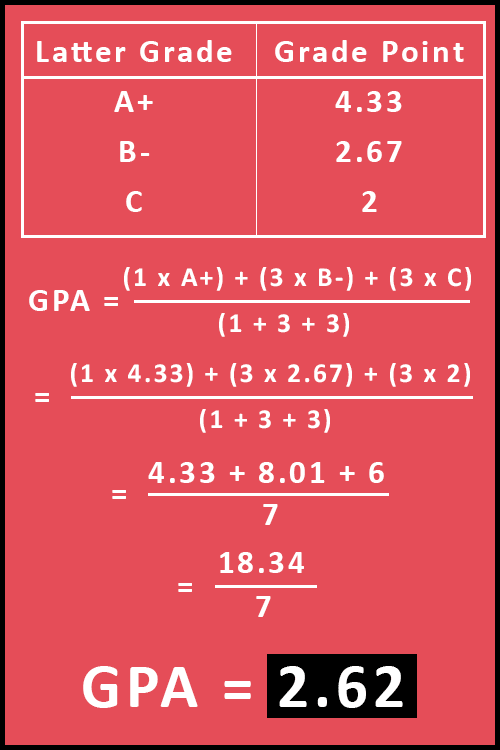Finally, we get GPA = 2.62.

Now we are clear with How to Calculate GPA. Just perform simple multiplication, addition, and division one by one. That's it.

But you don't need to do this manual calculation all the time. Because it's a very lengthy process. Even more, it will take more time when there are more than 7 or 8 courses. Instead of doing this, use our GPA calculator to save your time and effort. Also, our tool will give you 100% accurate output. Because it is working on pre-defined algorithms. Therefore, there is no chance for false results.

### Features of GPA Calculator

• Simple to use: The calculator is very easy to use just because of its simple and user-friendly interface. Just enter the values and calculate. That's it!
• Fast & 100% Accurate: Our tool provides you the final result in less than a second. Also, the web application is very lightweight. So, you can use it smoothly on low internet speed. In addition, the accuracy is 100%. There is a 0% chance to get false output.
• Save Time & Effort: It saves your time and effort as compared to manual calculation. Also, you don't need to remember the formula all the time.
• Add Multiple Courses: Our tool allows you to add an unlimited number of courses. You can add a new course using the "+ Add Course" button.
• No Need to login or Register: No login or signup required to use our tool. Just open it and start using it online.
• Supports all Browsers: We have made our College GPA Calculator all browsers compatible. So, it works smoothly on almost all web browsers.
• Mobile or iPad Friendly: Our tool is mobile and iPad responsive. Therefore, you can use it on any handy device anytime and anywhere.

#### How to use the College GPA Calculator?

It's very simple to use the tool. Just follow the steps below to get started.

##### 1. Enter course name (Optional)

Firstly, enter the course name (i.e. Maths, Physics, Chemistry, etc...) in the first column. It's an optional step. But don't skip it. We recommend you enter the course name from the beginning. Because it will help you to differentiate the exam grades and credit hours.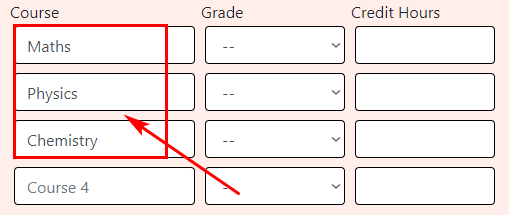Next step is to select the letter grades from A+ to NP. It's a second column where you can find these selection options.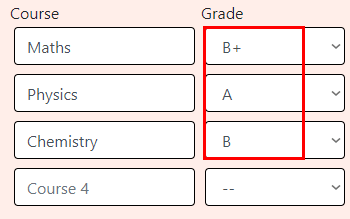##### 3. Enter Credit Hours

It is the most important step. In this step, you have to enter the credit hours that you have earned from each course.

Let's take an example to understand it.

If you earned B+ for 4 credit hour course, then you need to enter 4 in the third column box.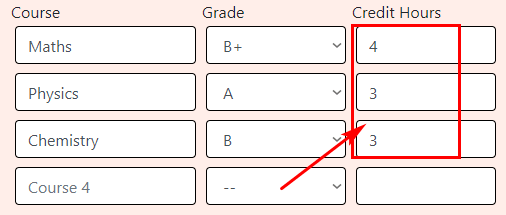If you are calculating GPA for less than 4 courses. Then it's Ok! But if you want to add more courses then you can use the "+ Add Course" button to add more rows. Also, you can add as many courses as you want. There is no limit on that.Even more, there is an optional field to enter your previous semester's cumulative GPA and Credits. You can enter these values if you want to find an average of an overall (Previous + Current) semester GPA.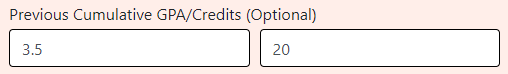##### 4. Calculate

Lastly, press the "Calculate" button to start the calculation process. Also, the "Reset" button will refresh the calculator.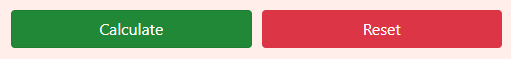##### 5. Get Results

Finally, you will get the grade point average, total credit hours, and full GPA calculation below.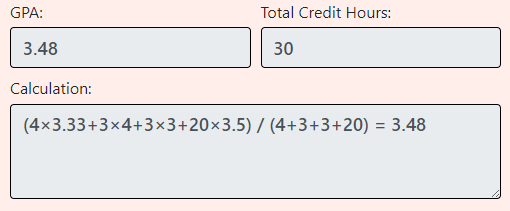### FAQs

To convert percentage into a 4.0 GPA, you can use the below formula.

GPA = ( x / 20 ) - 1

Where, x = Percentage of score

Let's take an example.

##### Example

A student gets 75% of the score in the History course in his final exam. Convert the percentage score into GPA.

Here, x = 75%

GPA = ( x / 20 ) - 1 = ( 75 / 20 ) - 1 = 3.75 - 1 = 2.75

Hence, we can say that the student got 2.75 GPA in his final exam.

You can add an infinite number of courses to the calculator. There is no specific limit for that.